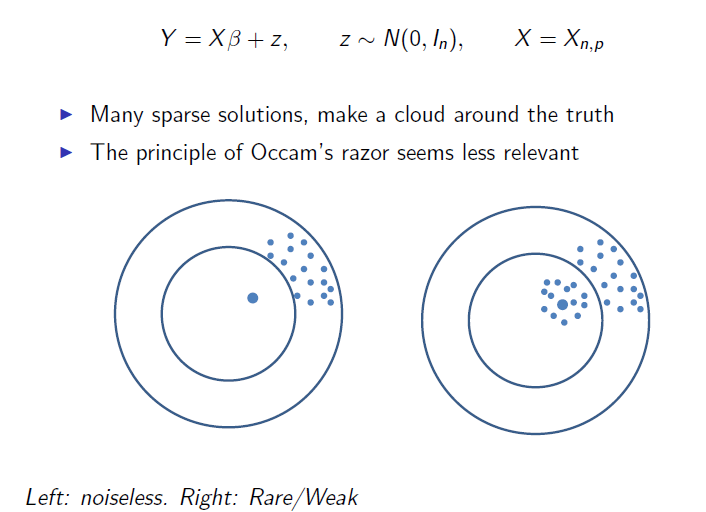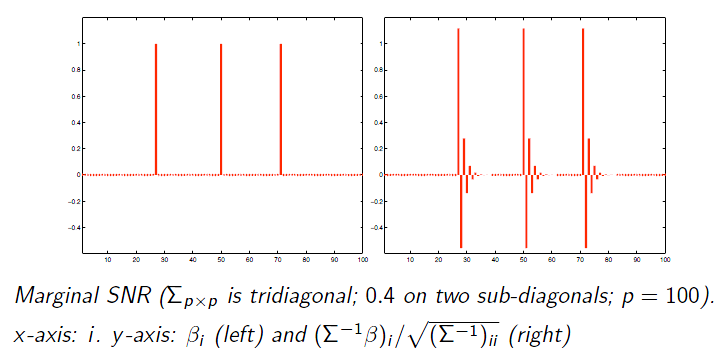# 前言

Surprisingly, penalization methods (e.g., the L0-penalization method) are not optimal for rare/weak signals, even in very simple settings and even with the tuning parameters ideally set。

## Donoho的不确定原则（Uncertainty Principle）与信号恢复

Jiashun老师说，关于信号恢复最早应该可以追溯到Donoho在1989年的论文Uncertainty Principle and Signal Recovery。在这片文章中，Donoho给出了类似于海森堡测不准原理的不确定原则（UP）。海森堡测不准原理通俗来讲即微观粒子某些物理量不可能同时被精确测量准确，一个量越确定，另外一个量的不确定程度就越大。Donoho的不确定原则通俗来讲即，离散时间点 $t = 0, 1, \ldots, n - 1$ 有观测 $Y_t$，做傅里叶变换有

$T$$W$ 分别表示 $Y_t$$\hat{Y}_w$ 中的非零的位置，那么就会得到一个不确定原则，

• $T \subset \{1, 2, \ldots, n\}$, 为“时域”，
• $W \subset \{n+1, \ldots, 2n\}$, 为“频域”，
• 要找到 $\beta$ 非零的位置，且具有稀疏性 $|T| + |W| \ll n$## Graphlet screening

Jiashun老师开头先讲了个故事，说有次Fan他们来他们那里做分享，很开心的分享他们关于screening、SIS等方面的工作，Jiashun老师自然先不了解Fan做的SIS和ISIS工作，对于screening也构想了自己的方法，但是当他听完Fan老师讲完他的思路后，他大腿一拍，“这不和我的思路一个样嘛！”

1. 相关性不是噩梦，对于估计可能会降低估计效率（相当于样本减少），但是对于信号检测，却是是一个福音。我举个例子来理解，比如舆情监测，在浩如烟海的网络上有个人喊了句“打到XX”，这信号弱的简直没人知道，但是如果有几个与这个人存在某种关系的人，他们也跟着喊了句“打到XX”，虽然都很弱，但是还是起到了增强信号的作用，相当于几个弱信号汇集一起，让其中一个人发出了怒吼“打到XX”，那么自然这个时候信号检测相对会容易些，而边上的几个弱信号也顺便被检测到。因此要善于利用相关性来增强信号，对于稀疏、弱信号是一个很重要的手段。
2. 信号稀疏，变量之间的相关性也是稀疏的。将变量间的相关性看做一个图，也就是说信号会分散到很多小子图中，而且这些小子图内部相关性强，而相互之间没什么联系。用形象的话来说，就是信号分散于很多小岛屿上，具体哪些小岛屿有信号还不太清楚，但是我知道信号就分散这些小岛上，小岛上处处散发着信号的微光，而小岛屿之间对另外一个信号的发现没有任何帮助。好比中国大地星星之火隐约可见，却不知他们各自藏身于何处。Jiashun老师对变量间的相关性用一个阈值来控制（screening），以达到图的稀疏（sparse）和seperable（分离）的效果。我个人觉得这个假设有点强，将大图划分为多个独立的子图，这种方法略显有些暴力啊，你想这些星星之火间真的没有联系么？好吧，就当没有什么联系吧，因为我也没啥特别好的想法:(

1. 假设变量形成的图 $\mathcal{G}$ 是稀疏的，信号可分解为多个独立子图 $\mathcal{G}_s$（最大连通子图）。于是利用相关性对原来的观测进行变化，通过这种方式增强信号后对图$\mathcal{G}$ 进行screening，即可获取支撑集，然后分解为多个子 $\mathcal{G}_s$
2. 在这些子图上，再利用加惩罚的MLE方法来估计、拟合即可。

1. Univariate Penalized Screening(UPS)

Jiashun老师提出假设简单模型

UPS方法不是直接对X或Y做screening，而是对变化数据 $\tilde{Y} = X'Y$ 做 screening，这其实一种增强信号的处理，以便于挑选rare/weak信号，它与之前Jiashun老师提出 Innovated Higher Criticism 有很强的的关联，先阐述 Innovated HC 方法以便于理解，Innovated HC 也是一种对于 rare/weak 信号挑选比较合适阈值的方法。对于阈值选择问题：

• 直接的想法就是用上一次提到的HC方法，假设各个信号独立无相关性来挑选信号，即 $\beta \sim N(\mu, I_p)$。但是实际情况多数各种信号噪音间有相关性 $\Sigma \neq I_p$，因此直接用HC似乎不那么美好。
• 另外一种想法就是常见的Whitening方法，用 $\Sigma^{-1/2}\beta$ 线性变化使得变量相关性去掉 $\Sigma^{-1/2}\beta \sim N(\Sigma^{-1/2}\mu, I_p)$，然后再用HC方法来挑选。
• 还有一种想法就是所谓的Innovated HC，应用HC于如下变化 $\Sigma^{-1}\beta \sim N(\Sigma^{-1}\mu, \Sigma^{-1})$

• 纯HC：$\tau$;
• Whitening后HC：$[2/(1+\sqrt{1 - h^2})]\tau$;
• Innovated后HC：$[1/(1 - h^2)]\tau$2. Graphlet Screening（GS）

• 只考虑需要考虑的有价值的的子模型，贯穿GOSD的想法到screening和cleaning中，只关注内部有强相关的子图，即仅利用Gram matrix中的 $X$ 来大大削减所需要考虑的子模型个数；同时还能解决信号抵消的问题。

GOSD降低计算量是因为假设图是 $k$ -sparse的，这样就从原来的 $O(p^m)$ 降到了差不多 $O(k^m)$ 的级别。具体的定理证明可详见Jiashun老师的论文和slides，此处再稍微提下GS的算法过程：

• 先利用GOSD获取稀疏图，然后在该图上选取子模型，每个子模型的节点数$\mathcal{I}_0$不超过 $m$ 个，对这些子模型进行screening。由于每个节点于自身互通，于是最初的的子模型都是单节点。
• 然后初始化留存节点集 $\mathcal{U}_{p}^{\star}$，对每个子模型地节点进行检验，是否要选入该子模型中的部分变量。想法是如果$Y$在整个该子模型 $\mathcal{I}_0$ 变量上的投影的平方和，与在该子模型与留存节点集共有变量 $\hat{F}=\mathcal{I}_0 \cap \mathcal{U}^{\star}_p$ 上的投影平方和，两者的差值如果大于某个阈值（经验性），则将该子模型的变量与留存变量集的差集变量选入，更新留存变量集（其实是一种适应性卡方检验）。该步骤的直观理解是如果两者的共有变量解释能力所占比重比较小，感觉像噪音，则说明另外一部分变量在该子模型中很可能是信号。
• screening后剩下的变量分解到各个自己的子模型 中$\mathcal{I}_0$，对这些子模型分别做类似 $\ell_0$ 变量选择既可挑选各个子模型的有用信号了。下面模型中的系数还有个阈值限制，需要大于$v^{gs}$$P^{\mathcal{I}_0}$ 表示在子模型 $\mathcal{I}_0$ 变量上的投影。